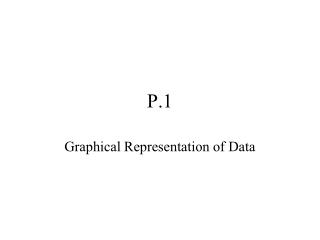DownloadDownload PresentationP.1

# P.1

Download Presentation## P.1

- - - - - - - - - - - - - - - - - - - - - - - - - - - E N D - - - - - - - - - - - - - - - - - - - - - - - - - - -
##### Presentation Transcript

1. P.1 Graphical Representation of Data

2. Renee Descartes “It is not enough to have a good mind. The main thing is to use it well.” • Born March 31, 1596 in La Haye Touraine, France • Died February 1, 1650 in Stockholm, Sweden

3. Philosopher whose work includes application of algebra to geometry • Entered college at the age of 8 • Poor health, permitted to sleep until 11 o’clock in the morning in college • Understood how little he knew • Only satisfactory subject was mathematics • Much (incorrect) work in meteorology “It is very certain that, when it is not in our power to determine what is true, we must act according to what is most probable.”

4. The Cartesian Plane “When writing about transcendental issues, be transcendentally clear.”

5. The Cartesian Plane Plot the following: (2,4) (-3,8) (7,-4) (-9,-6)

6. Representing Data Graphically In the table below, there are the average monthly temperatures for a small southern California town. Sketch the a scatter plot, a bar graph, and a line graph of the data.

7. Scatter Plot

8. Bar Graph

9. Line Graph “If you would be a real seeker of truth, you must at least once in your life doubt, as far as possible, all things.”

10. The Distance Formula

11. The Distance Formula Please find the distance between (2,-5) and (8,3). “I think, therefore I am.”

12. Please show that the points (1,-3), (3,2), and (-2,4) form an isosceles triangle. “With me, everything turns into mathematics.”

13. The Midpoint Formula

14. The Midpoint Formula • Find the midpoint of the line segment joining the points (-9,5) and (4,2).

15. The Equation of a Circle • Center: (h,k) • Radius: r

16. The Equation of a Circle • Find the standard form of the equation of the circle with center at (2,-5) and radius 4. “Each problem that I solved became a rule which served afterwards to solve other problems.”